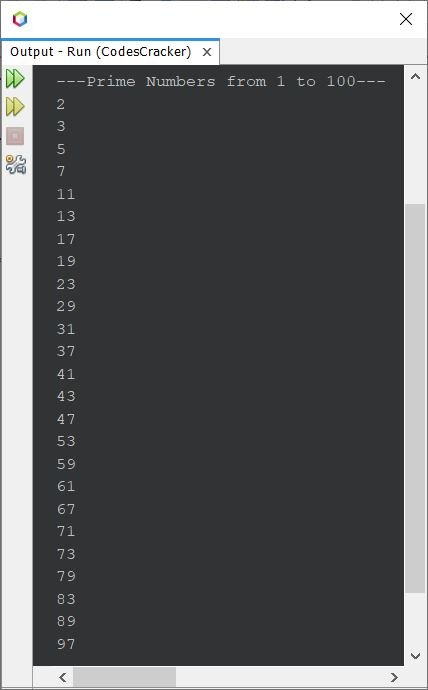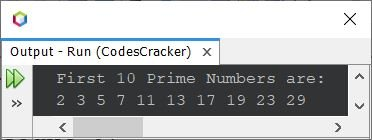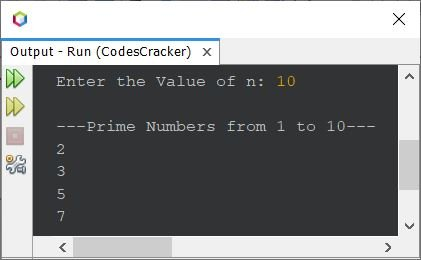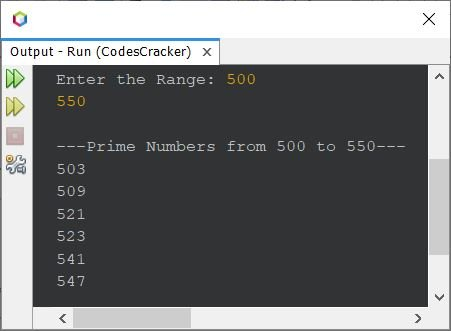# Java Program to Print Prime Numbers

• Print prime numbers from 1 to 100
• Print first 10 prime numbers
• Print prime numbers from 1 to n
• Print prime numbers in given range
• Print prime numbers using while loop

Note - A number that can only be divisible by 1 and the number itself is called a prime number. For example: 2, 3, 5, 7, 11, 13, 17 etc., are all prime numbers.

## Print Prime Numbers from 1 to 100 in Java

The question is, write a Java program to print prime numbers from 1 to 100. The program given below is its answer:

```public class CodesCracker
{
public static void main(String[] args)
{
int i, j, chk;

System.out.println("---Prime Numbers from 1 to 100---");
for(i=2; i<=100; i++)
{
chk = 0;
for(j=2; j<i; j++)
{
if(i%j==0)
{
chk++;
break;
}
}
if(chk==0)
System.out.println(i);
}
}
}```

The snapshot given below shows the sample output produced by above program, on printing of all prime numbers from 1 to 100:## Print First 10 Prime Numbers in Java

This program almost uses the same concept as of previous program, to print the first 10 prime numbers. In this program, I've used a variable named count, whose initial value is 0. And its value gets incremented each time by 1, after printing a prime number.

And the loop continue its execution, to print prime numbers, until the value of count becomes equal to 10, or until the condition count<10 evaluates to be False.

```public class CodesCracker
{
public static void main(String[] args)
{
int i, j, chk, count=0;

System.out.println("First 10 Prime Numbers are:");
for(i=2; count<10; i++)
{
chk = 0;
for(j=2; j<i; j++)
{
if(i%j==0)
{
chk++;
break;
}
}
if(chk==0)
{
System.out.print(i+ " ");
count++;
}
}
}
}```

The output produced by above program on printing of first 10 prime numbers, is shown in the snapshot given below:## Print Prime Numbers from 1 to n in Java

Now this program prints prime numbers from 1 to n. And of course, the value of n would be received by user at run-time of the program.

```import java.util.Scanner;

public class CodesCracker
{
public static void main(String[] args)
{
Scanner scan = new Scanner(System.in);

System.out.print("Enter the Value of n: ");
int n = scan.nextInt();

System.out.println("\n---Prime Numbers from 1 to " +n+ "---");
for(int i=2; i<=n; i++)
{
int chk = 0;
for(int j=2; j<i; j++)
{
if(i%j==0)
{
chk++;
break;
}
}
if(chk==0)
System.out.println(i);
}
}
}```

Here is its sample run with user input 10 as value of n to print prime numbers from 1 to 10## Print Prime Numbers in Given Range in Java

Now this program allows user to define the range, the start and end value, to print all prime numbers in between the given range.

```import java.util.Scanner;

public class CodesCracker
{
public static void main(String[] args)
{
Scanner scan = new Scanner(System.in);

System.out.print("Enter the Range: ");
int start = scan.nextInt();
int end = scan.nextInt();

System.out.println("\n---Prime Numbers from " +start+" to " +end+ "---");
for(int i=start; i<=end; i++)
{
int chk = 0;
for(int j=2; j<i; j++)
{
if(i%j==0)
{
chk++;
break;
}
}
if(chk==0)
System.out.println(i);
}
}
}```

The sample run with user input 500 and 550 as range, is shown in the snapshot given below:## Print Prime Numbers in Java using while Loop

The previous program can also be written using while loop, instead of for. For this, just replaced the for loop's block of code, with following block of code:

```int i=start;
while(i<=end)
{
int chk = 0;
int j=2;
while(j<i)
{
if(i%j==0)
{
chk++;
break;
}
j++;
}
if(chk==0)
System.out.println(i);
i++;
}```

#### Same Program in Other Languages

Java Online Test

« Previous Program Next Program »